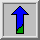# Hough Transform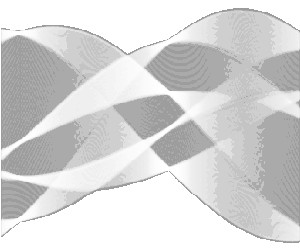Common Names: Hough transform

## Brief Description

The Hough transform is a technique which can be used to isolate features of a particular shape within an image. Because it requires that the desired features be specified in some parametric form, the classical Hough transform is most commonly used for the detection of regular curves such as lines, circles, ellipses, etc. A generalized Hough transform can be employed in applications where a simple analytic description of a feature(s) is not possible. Due to the computational complexity of the generalized Hough algorithm, we restrict the main focus of this discussion to the classical Hough transform. Despite its domain restrictions, the classical Hough transform (hereafter referred to without the classical prefix) retains many applications, as most manufactured parts (and many anatomical parts investigated in medical imagery) contain feature boundaries which can be described by regular curves. The main advantage of the Hough transform technique is that it is tolerant of gaps in feature boundary descriptions and is relatively unaffected by image noise.## How It Works

The Hough technique is particularly useful for computing a global description of a feature(s) (where the number of solution classes need not be known a priori), given (possibly noisy) local measurements. The motivating idea behind the Hough technique for line detection is that each input measurement (e.g. coordinate point) indicates its contribution to a globally consistent solution (e.g. the physical line which gave rise to that image point).

As a simple example, consider the common problem of fitting a set of line segments to a set of discrete image points (e.g. pixel locations output from an edge detector). Figure 1 shows some possible solutions to this problem. Here the lack of a priori knowledge about the number of desired line segments (and the ambiguity about what constitutes a line segment) render this problem under-constrained.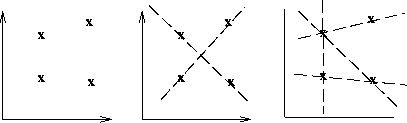Figure 1 a) Coordinate points. b) and c) Possible straight line fittings.

We can analytically describe a line segment in a number of forms. However, a convenient equation for describing a set of lines uses parametric or normal notion: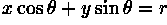where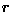is the length of a normal from the origin to this line and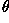is the orientation ofwith respect to the X-axis. (See Figure 2.) For any point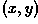on this line,andare constant.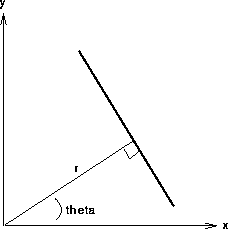Figure 2 Parametric description of a straight line.

In an image analysis context, the coordinates of the point(s) of edge segments (i.e.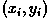) in the image are known and therefore serve as constants in the parametric line equation, whileandare the unknown variables we seek. If we plot the possible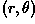values defined by each, points in cartesian image space map to curves (i.e. sinusoids) in the polar Hough parameter space. This point-to-curve transformation is the Hough transformation for straight lines. When viewed in Hough parameter space, points which are collinear in the cartesian image space become readily apparent as they yield curves which intersect at a commonpoint.

The transform is implemented by quantizing the Hough parameter space into finite intervals or accumulator cells. As the algorithm runs, eachis transformed into a discretizedcurve and the accumulator cells which lie along this curve are incremented. Resulting peaks in the accumulator array represent strong evidence that a corresponding straight line exists in the image.

We can use this same procedure to detect other features with analytical descriptions. For instance, in the case of circles, the parametric equation is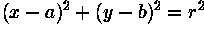where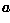and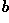are the coordinates of the center of the circle andis the radius. In this case, the computational complexity of the algorithm begins to increase as we now have three coordinates in the parameter space and a 3-D accumulator. (In general, the computation and the size of the accumulator array increase polynomially with the number of parameters. Thus, the basic Hough technique described here is only practical for simple curves.)

## Guidelines for Use

The Hough transform can be used to identify the parameter(s) of a curve which best fits a set of given edge points. This edge description is commonly obtained from a feature detecting operator such as the Roberts Cross, Sobel or Canny edge detector and may be noisy, i.e. it may contain multiple edge fragments corresponding to a single whole feature. Furthermore, as the output of an edge detector defines only where features are in an image, the work of the Hough transform is to determine both what the features are (i.e. to detect the feature(s) for which it has a parametric (or other) description) and how many of them exist in the image.

In order to illustrate the Hough transform in detail, we begin with the simple image of two occluding rectangles,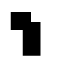The Canny edge detector can produce a set of boundary descriptions for this part, as shown in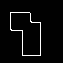Here we see the overall boundaries in the image, but this result tells us nothing about the identity (and quantity) of feature(s) within this boundary description. In this case, we can use the Hough (line detecting) transform to detect the eight separate straight lines segments of this image and thereby identify the true geometric structure of the subject.

If we use these edge/boundary points as input to the Hough transform, a curve is generated in polarspace for each edge point in cartesian space. The accumulator array, when viewed as an intensity image, looks like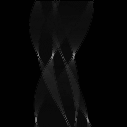Histogram equalizing the image allows us to see the patterns of information contained in the low intensity pixel values, as shown in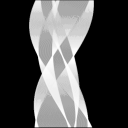Note that, althoughandare notionally polar coordinates, the accumulator space is plotted rectangularly withas the abscissa andas the ordinate. Note that the accumulator space wraps around at the vertical edge of the image such that, in fact, there are only 8 real peaks.

Curves generated by collinear points in the gradient image intersect in peaksin the Hough transform space. These intersection points characterize the straight line segments of the original image. There are a number of methods which one might employ to extract these bright points, or local maxima, from the accumulator array. For example, a simple method involves thresholding and then applying some thinning to the isolated clusters of bright spots in the accumulator array image. Here we use a relative threshold to extract the uniquepoints corresponding to each of the straight line edges in the original image. (In other words, we take only those local maxima in the accumulator array whose values are equal to or greater than some fixed percentage of the global maximum value.)

Mapping back from Hough transform space (i.e. de-Houghing) into cartesian space yields a set of line descriptions of the image subject. By overlaying this image on an inverted version of the original, we can confirm the result that the Hough transform found the 8 true sides of the two rectangles and thus revealed the underlying geometry of the occluded scene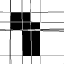Note that the accuracy of alignment of detected and original image lines, which is obviously not perfect in this simple example, is determined by the quantization of the accumulator array. (Also note that many of the image edges have several detected lines. This arises from having several nearby Hough-space peaks with similar line parameter values. Techniques exist for controlling this effect, but were not used here to illustrate the output of the standard Hough transform.)

Note also that the lines generated by the Hough transform are infinite in length. If we wish to identify the actual line segments which generated the transform parameters, further image analysis is required in order to see which portions of these infinitely long lines actually have points on them.

To illustrate the Hough technique's robustness to noise, the Canny edge description has been corrupted by 1% salt and pepper noise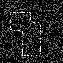before Hough transforming it. The result, plotted in Hough space, is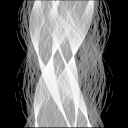De-Houghing this result (and overlaying it on the original) yields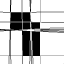(As in the above case, the relative threshold is 40%.)

The sensitivity of the Hough transform to gaps in the feature boundary can be investigated by transforming the image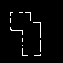, which has been edited using a paint program. The Hough representation is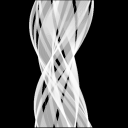and the de-Houghed image (using a relative threshold of 40%) is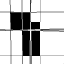In this case, because the accumulator space did not receive as many entries as in previous examples, only 7 peaks were found, but these are all structurally relevant lines.

We will now show some examples with natural imagery. In the first case, we have a city scene where the buildings are obstructed in fog,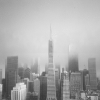If we want to find the true edges of the buildings, an edge detector (e.g. Canny) cannot recover this information very well, as shown in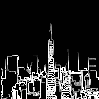However, the Hough transform can detect some of the straight lines representing building edges within the obstructed region. The histogram equalized accumulator space representation of the original image is shown in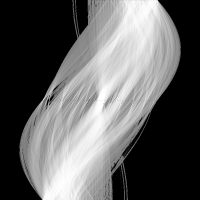If we set the relative threshold to 70%, we get the following de-Houghed image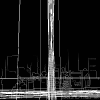Only a few of the long edges are detected here, and there is a lot of duplication where many lines or edge fragments are nearly colinear. Applying a more generous relative threshold, i.e. 50%, yields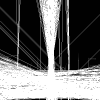yields more of the expected lines, but at the expense of many spurious lines arising from the many colinear edge fragments.

Our final example comes from a remote sensing application. Here we would like to detect the streets in the image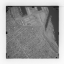of a reasonably rectangular city sector. We can edge detect the image using the Canny edge detector as shown in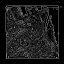However, street information is not available as output of the edge detector alone. The image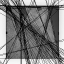shows that the Hough line detector is able to recover some of this information. Because the contrast in the original image is poor, a limited set of features (i.e. streets) is identified.

## Common Variants

Generalized Hough Transform

The generalized Hough transform is used when the shape of the feature that we wish to isolate does not have a simple analytic equation describing its boundary. In this case, instead of using a parametric equation of the curve, we use a look-up table to define the relationship between the boundary positions and orientations and the Hough parameters. (The look-up table values must be computed during a preliminary phase using a prototype shape.)

For example, suppose that we know the shape and orientation of the desired feature. (See Figure 3.) We can specify an arbitrary reference point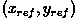within the feature, with respect to which the shape (i.e. the distanceand angle of normal lines drawn from the boundary to this reference point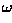) of the feature is defined. Our look-up table (i.e. R-table) will consist of these distance and direction pairs, indexed by the orientationof the boundary.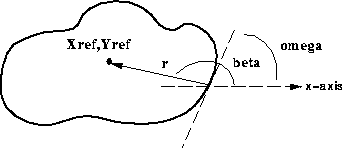Figure 3 Description of R-table components.

The Hough transform space is now defined in terms of the possible positions of the shape in the image, i.e. the possible ranges of. In other words, the transformation is defined by: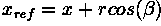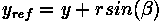(Theand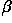values are derived from the R-table for particular known orientations.) If the orientation of the desired feature is unknown, this procedure is complicated by the fact that we must extend the accumulator by incorporating an extra parameter to account for changes in orientation.

## Interactive Experimentation

You can interactively experiment with this operator by clicking here.

## Exercises

1. Find the Hough line transform of the objects shown in Figure 4.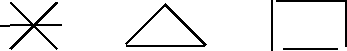Figure 4 Features to input to the Hough transform line detector.

2. Starting from the basic imagecreate a series of images with which you can investigate the ability of the Hough line detector to extract occluded features. For example, begin using translation and image addition to create an image containing the original image overlapped by a translated copy of that image. Next, use edge detection to obtain a boundary description of your subject. Finally, apply the Hough algorithm to recover the geometries of the occluded features.

3. Investigate the robustness of the Hough algorithm to image noise. Starting from an edge detected version of the basic imagetry the following: a) Generate a series of boundary descriptions of the image using different levels of Gaussian noise. How noisy (i.e. broken) does the edge description have to be before Hough is unable to detect the original geometric structure of the scene? b) Corrode the boundary descriptions with different levels of salt and pepper noise. At what point does the combination of broken edges and added intensity spikes render the Hough line detector useless?

4. Try the Hough transform line detector on the images: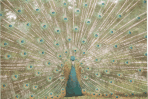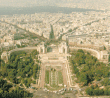and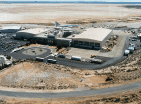Experiment with the Hough circle detector on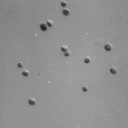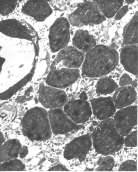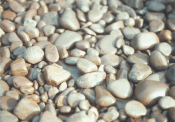and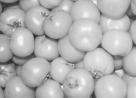5. One way of reducing the computation required to perform the Hough transform is to make use of gradient information which is often available as output from an edge detector. In the case of the Hough circle detector, the edge gradient tells us in which direction a circle must lie from a given edge coordinate point. (See Figure 5.)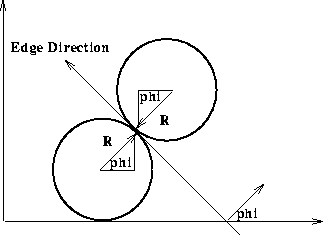Figure 5 Hough circle detection with gradient information.

a) Describe how you would modify the 3-D circle detector accumulator array in order to take this information into account. b) To this algorithm we may want to add gradient magnitude information. Suggest how to introduce weighted incrementing of the accumulator.

6. The Hough transform can be seen as an efficient implementation of a generalized matched filter strategy. In other words, if we created a template composed of a circle of 1's (at a fixed) and 0's everywhere else in the image, then we could convolve it with the gradient image to yield an accumulator array-like description of all the circles of radiusin the image. Show formally that the basic Hough transform (i.e. the algorithm with no use of gradient direction information) is equivalent to template matching.

7. Explain how to use the generalized Hough transform to detect octagons.

## References

D. Ballard and C. Brown Computer Vision, Prentice-Hall, 1982, Chap. 4.

R. Boyle and R. Thomas Computer Vision:A First Course, Blackwell Scientific Publications, 1988, Chap. 5.

A. Jain Fundamentals of Digital Image Processing, Prentice-Hall, 1989, Chap. 9.

D. Vernon Machine Vision, Prentice-Hall, 1991, Chap. 6.

## Local Information